Confidence IntervalsNext: Parameter Recovery Up: Simulations Previous: Simulations

Confidence Intervals

The validity of the confidence intervals returned by the Bayesian approach in ELFIT2D can be tested by running ELFIT2D on a number of noise realizations of the same galaxy rotation curve spectrum. A simulation of a typical SIS/LORAL3 spectrum (t = 2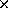3600sec, N= 8 e) of a z = 0.35 galaxy with Vsin= 130 km/s, disk scale length of 2.0hkpc, [OII] ratio of 1.0, total [OII] flux of 1053 DU and background level of 0 DU was created. Random noise was added to this galaxy spectrum using the IRAF task MKNOISE in the ARTDATA package. Fifty galaxy spectra were created using a different seed for the noise each time. ELFIT2D was run with the same initial conditions on all fifty spectra. The results are given in Tableand shown in Figure.

Columns 2-5 in Tableshow that the measured median values are scattered in an unbiased way around the true parameter values. Column 8 gives for each parameter the fraction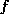of spectra in which the true parameter value was within the 68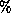confidence interval derived by ELFIT2D from the posterior probability distribution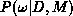. These confidence intervals include the true value between 0.56 and 0.72 of the time according to thevalues. The expected value of 0.68 forcorresponds to 34 spectra out of 50, and the= 5.8 Poisson error can account for the fluctuations seen in. Therefore, the confidence intervals given by ELFIT2D are valid. Figureshows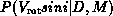for three noise realizations. It is interesting to note that the skewness of the distributions exhibits the same behavior as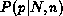in the suspicious coin experiment (see Figure).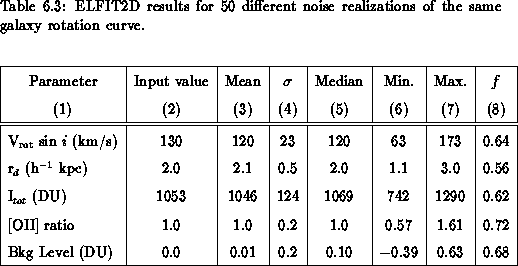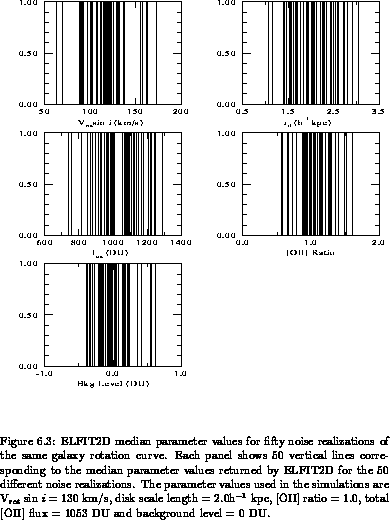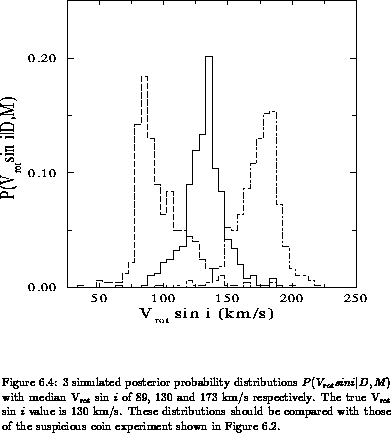Luc Simard
Mon Sep 2 12:37:40 PDT 1996